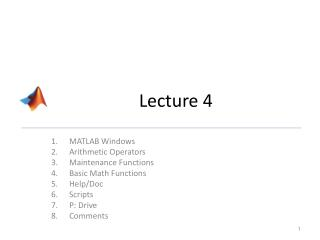DownloadDownload PresentationLecture 4

# Lecture 4

Download Presentation## Lecture 4

- - - - - - - - - - - - - - - - - - - - - - - - - - - E N D - - - - - - - - - - - - - - - - - - - - - - - - - - -
##### Presentation Transcript

1. Lecture 4 MATLAB Windows Arithmetic Operators Maintenance Functions Basic Math Functions Help/Doc Scripts P: Drive Comments

2. Fundamental components of MATLAB • MATLAB stands for Matrix Laboratory • MATLAB = a calculator + a lot more • A computing system that interprets one instruction at a time. Instructions MUST conform to a specific syntax and vocabulary. • A large library of modules that provide high-level capabilities for processing data. • A collection of toolboxes, some providing graphical capabilities. • A graphical user interface (GUI) to allow you to assemble your own professional looking software.

3. Starting MATLAB • Opening MATLAB in LB172: computer should have a direct shortcut on the desktop • Any other LAB on campus, search: • If not, use Ernie (directions online) Double Click to bring up the MATLAB Interface

4. DONOT USE CITRIX IN THE LAB • Why? …

5. “Workspace” “The Command Window” “Current Directory” Shows the variables that have been created. “Your Calculator Screen” You can do exactly as on your calculator: add, subtract, divide, multiple, use parentheses to override the order of operations… Later on, you will realize you can do a LOT more in this frame. This frame shows the files (excel, text, MATLAB files) that can immediately be used. “Command History” It will show all the commands executed previously. None of these are helpful to write re-usable code.

6. 1. Basic Data Manipulation • Starting MATLAB leads to the prompt symbols>> in the command window • If you don’t see the prompt symbols, MATLAB is busy MATLAB is loading MATLAB is doing 1,000,000 calculations… MATLAB has crashed MATLAB is stuck

7. Overview: most Operators • There are 3 primary groups of operators • One operator is very different in programming compared to math

8. MATLAB as a Calculator • The MATLAB command window can be used as a boring old calculator. • Precedence is similar to most calculators with a few MATLAB specific things. Left to right based on these things: 8

9. MATLAB as a Calculator • Mistake you’ll make at least once! • Power is before signage: -3^2 is different than (-3)^2 9

10. Demo MATLAB as a calculator in the command window (without variables) • Any single line contains a command/statement/instruction (different words for the same concept) • The ans variable is the default location where MATLAB stores results.

11. Maintenance Functions • clear • clc • close all • commandwindow

12. Basic Math Functions • sin • cos • tan • sind • sqrt • abs • help vs. doc • help elfun

13. the “Editor” • “Open a new script file"

14. A new window: the Editor This is where you’ll create a “script file” Save these type of files (on your P:/ drive )  absolutely re-usable

15. A new window: the Editor “Dock” this window to make it part of the regular windows below